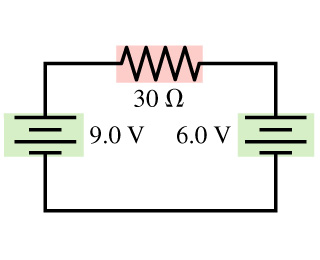# Problem: Part A. What is the magnitude of the current in the 30-Ω resistor in the figure? Answer in AmperesPart B. What is the direction of the current?

###### FREE Expert Solution

Ohm's law:

$\overline{){\mathbf{V}}{\mathbf{=}}{\mathbf{i}}{\mathbf{R}}}$

(a)

Let us assume the current move in a clockwise direction.

Applying Kirchoff's loop rule:

9.0V - i(30Ω) - 6.0V = 0

99% (410 ratings)###### Problem DetailsPart A. What is the magnitude of the current in the 30-Ω resistor in the figure? Answer in Amperes

Part B. What is the direction of the current?